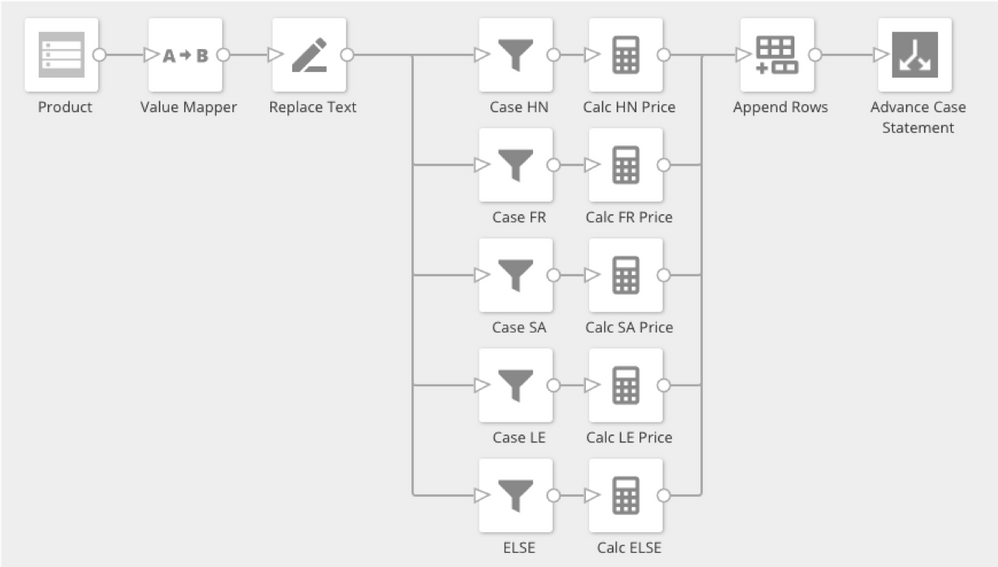# Is there a way to do this in Magic ETL?ContributorI've got the following CASE statement in a beast mode now, wondering if there is a way I can do in Magic ETL so I can then use in card filters.

CASE
WHEN SUM(`Order Quantity`) > SUM(`Quantity Available`) THEN SUM(`Value Available`)
ELSE SUM(`Order Value at 02 Slow Item Cost`)
END

•CoachHow are you wanting your card filter to behave? It's possible you may not need to do an ETL.

•ContributorFor example, would want to filter where the result is >\$5000 only.

Regardless, would like to know how to do this in Magic ETL if possible.

Thank you

•CoachHopefully someone else can chime in on the ETL portion. But you can create the filter by creating another BeastMode

`CASE WHEN CASE WHEN SUM(`Order Quantity`) > SUM(`Quantity Available`) THEN SUM(`Value Available`)ELSE SUM(`Order Value at 02 Slow Item Cost`)END> 5000 THEN '> 5k'ELSE '< 5k'END`

That would let you filter between > 5k or < 5k.

•ContributorThanks @Valiant, I appreciate the BeastMode help.

Still hoping for some ideas on the Magic ETL.

• Ever get an answer to this?  Looking for the same thing.

• It turns out that this question is a little trickier than it may seem on the surface. Any time we are using an aggregate function (SUM, in this case) there are decisions that need to be made about how the data is being grouped and filtered in order to give you that aggregation.

For example, in the original formula that was provided:

CASE
WHEN SUM(`Order Quantity`) > SUM(`Quantity Available`) THEN SUM(`Value Available`)
ELSE SUM(`Order Value at 02 Slow Item Cost`)
END

There is an assumption that the data is being grouped by item, time frame, etc., and may be filtered by time frame, region, warehouse, etc.

If those groupings and filters are going to be hard-coded into the setup of the card, then we can use a dataflow with matching groupings and filters to do what you're after. If the groupings or filters will change on the fly, then it will no longer make sense to create the dataflow to accomplish this.

Taking the idea a little further, if the grouping and filtering are set and won't be changed, then it probably make sense to use a dataflow to create a dataset that is already filtered and aggregated to that level. Then the SUM functions wouldn't be needed in the beast mode and it would work to use it as a filter.

There is a post in the ideas exchange (link below) regarding adding aggregate filters to the Analyzer. I'd recommend upvoting this idea and providing feedback regarding the need for this type of filtering.

I hope that helps!

• I agree with ZCameron, you need to ask if putting the aggregate in a DataFlow is the correct place or not.

I would also suggest you go like the Idea to get it added to the product.

To answer your question in the basics, you can do a case statement in Magic. It isn't very pretty, but it works. See image for an example:Case Statement using Filters

You simply create a Filter for each of the case statement, then do the aggregate then append them back.

So here you see a Filter for HN, FR, SA, and LE. Then you see an Else Filter to catch anything else. So the Case statement will look this:

`CaseWhen CountryCode = 'HN' then Sum(Price)When CountryCode = 'FR' then Sum(Price)When CountryCode = 'SA' then Sum(Price)When CountryCode = 'LE' then Sum(Price) Else Sum(Price)End`

The Sum(Price) can be any functions you need to take care of.

Remember to think of the Else statement and make sure you don't overlap in your Case Filters.

Once you have down the calculations on the filtered data, you just append it back together.